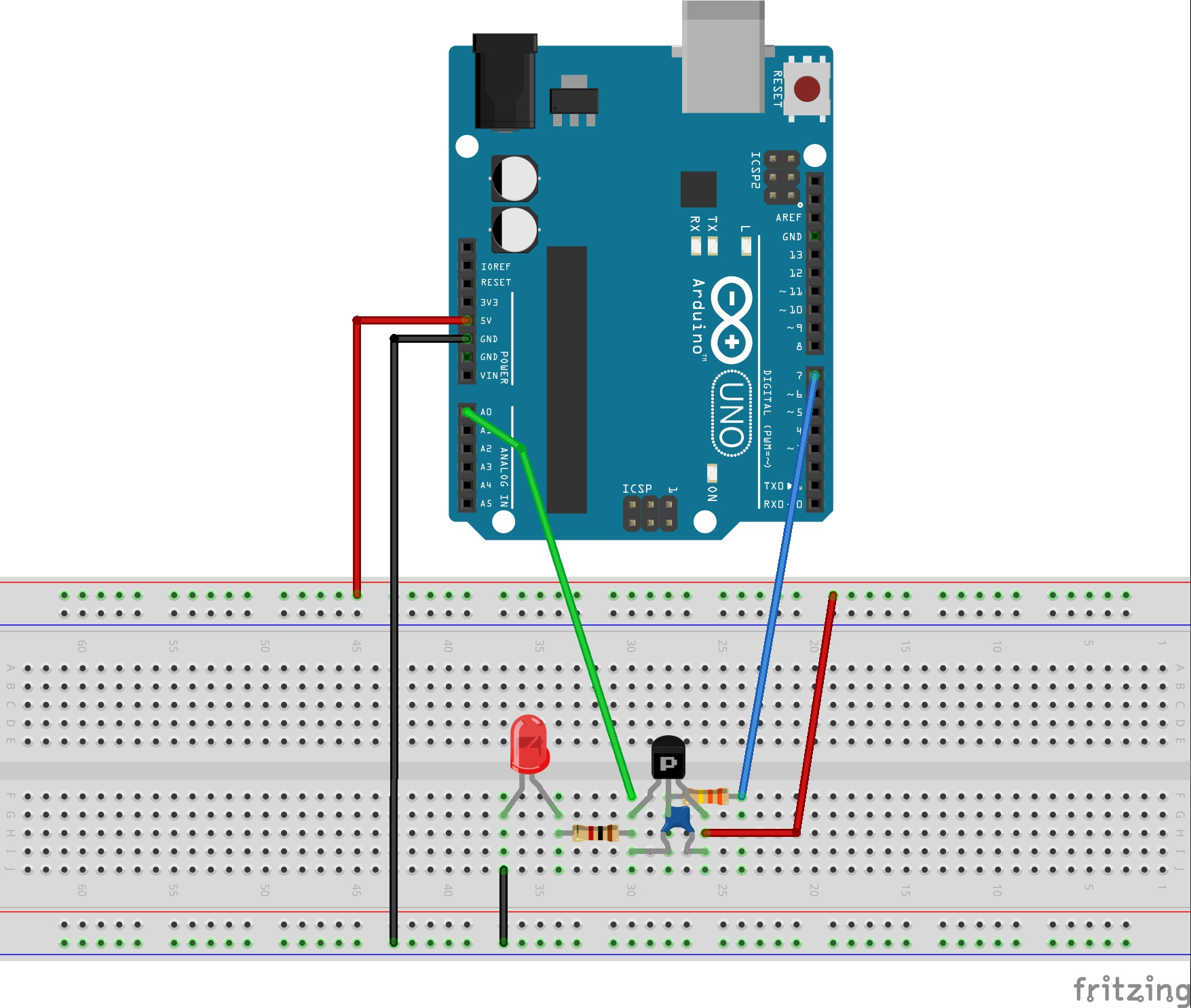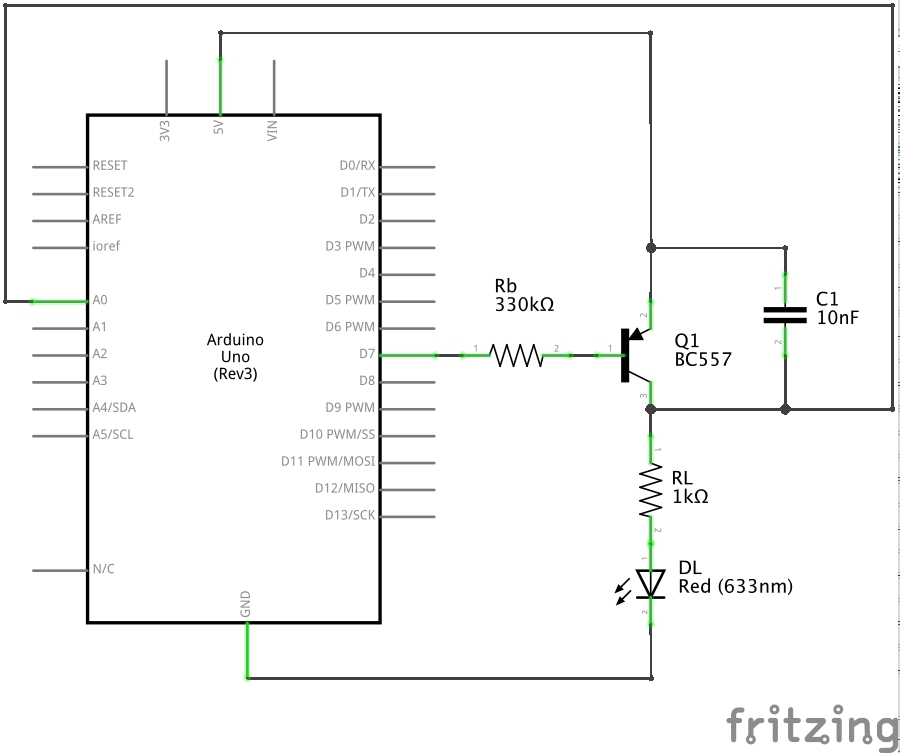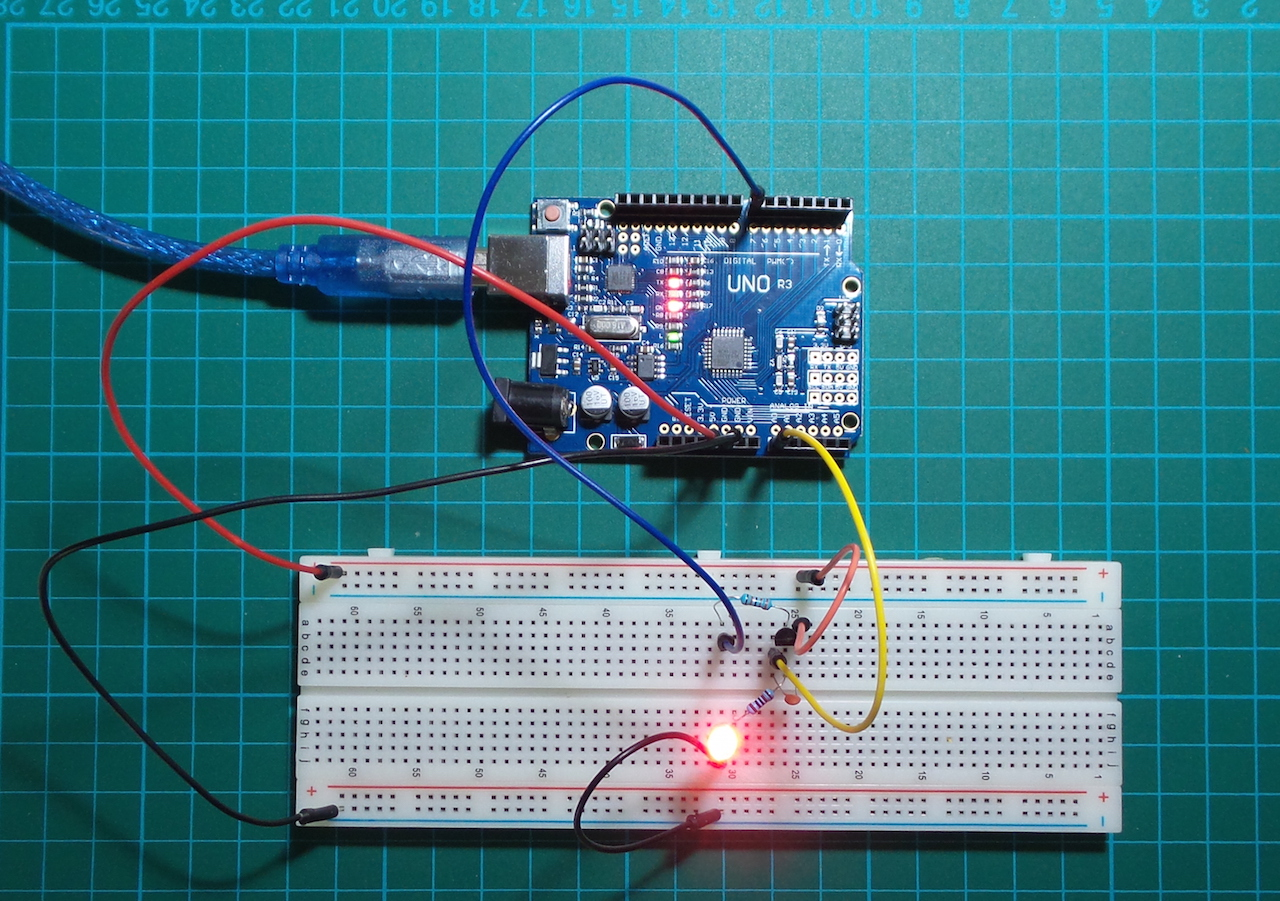# #033 Digital Logic Switch - PNP

Use an Arduino to demonstrate a digital switch using an PNP BJT, and plot the results with Processing.

This is a demonstration a standard small-signal switching circuit using an PNP transistor.

A digital out pin is used to flip the switch by sending the base of the transistor alternately high and low.

The BC557 transistor used here is rated for 100mA max, so only a small (LED) load is connected. Designing for a 2mA current, the current gain is 220 and thus the expected current to the base (Ib) is 9uA.

At Ib=9uA and Vbe=0.7V, desired Rb is:

``````Rb = (Vin - Vbe) / Ib = (5 - 0.7)/ 9x10^-6 = 478kΩ
``````

Looking at my parts box, I picked 330kΩ instead. Actual current measurements with the switch ON:

Ref Design Measured
Ic 2mA 2.85mA
Ib 9uA 12uA

Aside from the LED, an analog input pin is used to read a voltage in the load chain to see what is going on and send the data to PlotNValues (a simple Processing sketch) for plotting over time.

Here’s a sample trace. It also illustrates the impact of the filter capacitor C1 - the first 5 cycles in the plot are without C1, the remainder with C1 in place.### Construction